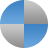# Intro Physics

## E = mc2

### Curriculum & Classroom

Rules & Expectations PPT| Rules and Expectations .doc

Multiple Intelligences Test

### The Nature of Science

Mystery Eggs (opening experiment)Powerpoint Lecture: Scientific Method | Lecture NotesPowerpoint Lecture: Standards of Measurement | Lecture NotesPowerpoint Lecture: Interpreting & Creating Graphs | NotesMath & Physics | Lecture Notes

### MotionPowerpoint Lecture: Motion | Lecture Notes

Problemset: Distance & Displacement
Problemset: Velocity

Problem Set: Acceleration

Review Guide: Motion

#### ForcesPPT: Motion & Forces (Newton's Law) | Lecture NotesPPT: Newton's 2nd Law (F=ma) | Lecture Notes

FunLab: Design a Balloon Car

Problemset: Force and Acceleration (F=ma)
Problemset: Mass and Weight  | MomentumPowerpoint Lecture: Newton's Third Law (collisions)
Lecture Notes

Activity: Design a website for Isaac Newton

Review Guide: Newton's 2nd and 3rd Laws

### EnergyPowerpoint Lecture: Potential & Kinetic Energy | Lecture Notes

Article: Wicked Rollercoaster

Elab: Coaster Crafter

Problem Set: Kinetic and Potential Energy

Review Guide: Energy

### WorkPowerpoint Lecture: Work and Power | Student NotesPowerpoint Lecture: Simple Machines | Student Copy

Work and Machines: Vocabulary

(Lab) Lever Lab: Calculate Mechanical Advantage
(Lab) Pulleys and Inclined Planes: If Your Need to Move a Piano.

(ELab) Pulley Systems

For Fun: Build a system of gears with Gearsket.ch

### Electricity & MagnetismPowerpoint Lecture: Electricity | Student NotesPowerpoint Lecture: Magnets | Student Notes

Electricity ProblemSet (Ohm's Law)

### WavesPowerpoint Lecture: Waves | Lecture NotesPowerpoint Lecture: Sound (Waves) | Lecture NotesPowerpoint Lecture: Electromagnetic Waves | Lecture NotesPowerpoint Lecture: Light & Color | Lecture Notes

Final Project: Technology and Inventions

Final Exam Practice Quizzes: Scientific Method & Motion | Forces | Energy & Work | Electricity & Magnets |
Final Exam Topic Review Guide | Final Exam Fill in the Blank Review

If it's green, it's BIOLOGY. ▪ If it stinks, it's CHEMISTRY. ▪ If it doesn't work, it's PHYSICS

##### Lab Safety Guidelines

Students will be doing many laboratory activities that may require the use of chemicals, laboratory equipment, and other items which, if used incorrectly, can be hazardous.

##### Problemset: Acceleration

Practice the acceleration equation by identifying variables: final velocity, initial velocity, and time.

##### Wicked at Lagoon

How do roller coasters use potential and kinetic energy to design loops and hills. (Literacy and Science)

##### Balancing Act - Lever Lab

Learn how the arm length can affect the amount of work done by a lever in this virtual lab by PHET.

##### Circuit Construction Kit

Construct circuits and run tests to determine how voltage and resistance affects the brightness of a bulb.

##### Ohm's Law

This problemset uses the Ohm's Law equation to calculate voltage, resistance, and current within a circuit.

##### Magnets

This article gives a brief overview of how magnets are used in everyday living.